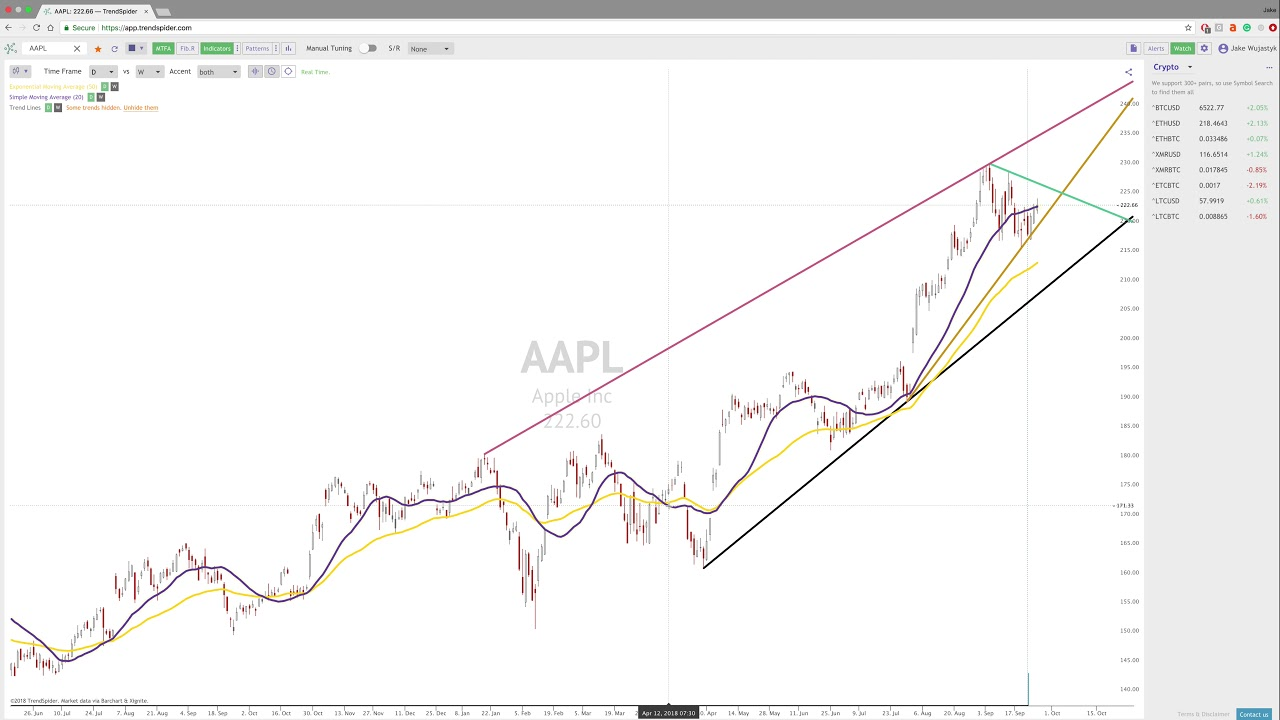09/26/2018 |

# Trading the SMA vs. the EMA

When most traders think of moving average confirmation strategies, they may immediately think of the generic and most widely used ones such as the 50-day Simple Moving Average vs. the 200-day Simple Moving Average. However, even though these can be very useful, especially as many traders become educated and are looking at the same thing creating self-fulfilling moves, there are other moving average relationships that backtest very well.

## The Simple Moving Average vs. the Exponential Moving Average

The “Simple Moving Average” is a slower indicator that has a mathematical formula that is simply the mean of closing prices over X period of time plotted on a chart. In this case, every single close within the equation has equal weight (i.e. for a 20-day moving average, you would add each of the last 20 closes and divide that number by 20, giving each close a 5% weight).

### Simple Moving Average Equation### “Smoothing Factor” Equation

The “Exponential Moving Average” is still a moving average that is plotted on the chart but is more sensitive to recent price action than the “Simple Moving Average”. In this case, the weight is added to the most recent price action through the “Smoothing Factor” which is shown in the figure below.### Exponential Moving Average EquationAs you can see, the equation for the “Exponential Moving Average” is much more complex than calculating the “Simple Moving Average” as it adds a level of complexity to give the more recent action more weight. Some traders like using this combination as it shows the relationship between the general price action over a specific amount of time vs. the most recent price action.The relationship between the SMA and EMA when they are both on equal inputs (in this case we used twenty (20) 30-minute time frames).